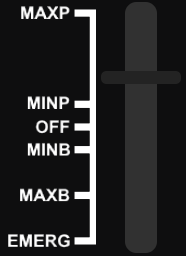# [SOLVED] Help with correct throttle percentage

Hello, I am working on this throttle for a vehicle but I cannot get the percentages right when the throttle handle moves up or down.

At MaxP / when Position.Y.Scale is 0 I would like it to print out 100% and when at MinP / when Position.Y.Scale is 0.4 then I would like it to print out 1% and when Position.Y.Scale is 0.2 I would like to print out 50%.

So when the throttle handle moves up I would like to increase it by 25% and when the throttle hand moves down I would like to decrease it by 25%. There is a distance of 0.1 each time the handle moves and each of them is 25%.

So basically,
0 = 100%
0.1 = 75%
0.2 = 50%
0.3 = 25%
0.4 = 1%
0.5 = 0%
0.6 = -1%
0.7 = -50%
0.8 = -100%
0.9 = -105%
1 = -110

Currently there is a function that checks the scale and returns a number but I think that way looks ugly and not good.Thanks anyone for any help! I’m not very good at math so I can’t really do it.

Except that 25% doesn’t equal .1 each time.
If you have 210% total travel and want each percent increment equal then each equal increment would have to be 21% so using math to calculate it doesn’t work the way you’d like it to.
For your system it would be better just to have your throttle script set each of those 21% increments to whatever power level you require.
Basically use the table you gave and when you increase from 0 to 1% change the scale of your GUI to .4.
If the reverse power is ‘increased’ from -1 to -50% then change the scale to .7.

Hold on ignore between off and emerge and lets just use minp and maxp for a now everytime you increase the throttle from minp it increases by 0.1 how could I get a percentage between each one of those that increases by 25%? This could work.

I’ve tried to go 0.1 * 25 * 10 which would give 25% instead of 75% so it kind of works but its kind of backwards if you know what I mean and for the same if 0.1 is a different Y scale and with below Off I might change that to decrease by 25% each time is moves down.

The problem as I see it is that you are trying to make a 0-1 scaled item work mathematically with a +100% to a -100% (for example) scaled item.

Make your power reference value (I don’t know what your thrust is, but let’s use a a VectorForce in this example. Of course you’ll have to change the step increments for whatever you require.

It takes +1000 force to drive your vehicle and -1000 to go full reverse.
Each time you change throttle you want a 25% change in force.
I just wrote the calculations similar to how coding it would work
forceMax = 1000
forceMin = 1000
force = the actual amount driving the vehicle
scale = the amount the GUI is set at (100% = 0 and -100% = 1)

``````get input
if input is increase (lets say W) and force is <= forceMax then
force += (.25 * maxForce) -- increases the force pushing the vehicle  by 25% per step
scale = 1 - ((force + maxForce) / maxForce) * .5)  -- 1000 would be scale 0, 500 would be scale.25, 0 would be scale .5, -1000 would be scale 1
elseif input is decrease (say S) and force >= -forceMax then
force -= (.25 * maxForce) --decreases by 25%
scale = 1 - ((force + maxForce) / maxForce) * 2)  -- same calculation as above, but we are decreasing the force with the input.
end
``````

Sorry for the month late reply!

But I’ve figured out a better way and less confusing way. I’ll edit this post tomorrow with the solution I did.